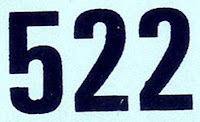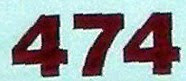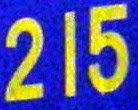## Friday, October 29, 2010

### 262

262 = 2 x 131. It is the product of two distinct Sophie Germain primes.

262 is a meandric number: the number of ways a river can cross a road n times.

The sum of the fourth powers of the prime factors of 262 is a prime: 24 + 1314 = 294499937, a prime.

The concatenation of the proper divisors (excluding 1 and the number itself) of 262 is a prime: 2131 .The Messerschmitt Me 262 was the world's first operational jet-powered fighter aircraft.

Source: Wikipedia

## Thursday, October 28, 2010

### 55

55 = 5 x 11.

55 is the tenth Fibonacci number.

55 is the tenth triangular number.

55 is a pyramidal number, the sum of the first five square numbers: 55 = 12 + 22 + 32 + 42 + 52.

55 is a number that cannot be written as a sum of three squares.An emerald anniversary celebrates 55 years.

Source: Phillips, R. 2004. Numbers: Facts, Figures and Fiction. Badsey Publications.

## Wednesday, October 27, 2010

### 140

140 = 22 x 5 x 7.

140 has a unique representation as a sum of three squares: 140 = 22 + 62 + 102.

140 is a square pyramidal number. It is the sum of the first seven squares: 140 = 12 + 22 + 32 + 42 + 52 + 62 + 72.140 Siwa is a large, dark asteroid discovered in 1874.

## Tuesday, October 26, 2010

### 434

434 = 2 x 7 x 31.

434 is a palindrome that produces a composed prime: 42 + 37 + 431 = 4611686018427390107, a prime.

434 is the smallest composite number that satisfies the equation sigma(n + 2) = sigma(n) + 2, where sigma(n) is the sum of the divisors of n.

434 is 2002 in base 6.434 Hungaria is an asteroid discovered in 1898.

## Monday, October 25, 2010

### 859

859 is a prime number.

857 and 859 form a twin prime pair.

859 is the largest prime that cannot be the central number in a 3-by-3 magic square.

859 is the sum of nine positive fifth powers: 859 = 15 + 15 + 25 + 25 + 25 + 25 + 35 + 35 + 35.859 Bouzar

# é

ah is an asteroid discovered in 1916.

## Friday, October 22, 2010

### 217

217 = 7 x 31.

47 and 89 are primes, as is their concatenation: 4789. Inserting 0 between them transforms the prime into a power: 47089 = 2172.

217 is 1001 in base 6 and 161 in base 12.217 is the telephone area code covering much of central Illinois.

## Thursday, October 21, 2010

### 64

64 = 26.

64 is the first number after 1 to be both a square and a cube: 64 = 82 = 43.

64 is the number of integer partitions of 20 into distinct parts.

64 is 121 in base 7 and 44 in base 15. It is 1000000 in base 2, 1000 in base 4, 100 in base 8, 40 in base 16, and 20 in base 32.The Commodore 64 was an 8-bit home computer introduced in 1982.

Source: Phillips, R. 1994. Number Facts, Figures and Fiction. Cambridge University Press.

## Wednesday, October 20, 2010

### 522

522 = 2 x 32 x 29.

522 has a representation as a sum of two squares: 522 = 92 + 212.

522 is the sum of six consecutive primes: 522 = 73 + 79 + 83 + 898 + 97 + 101.

522 is the sum of seven positive fifth powers: 522 = 15 + 15 + 15 + 15 + 25 + 35 + 35.The SOKO 522 was a two-seater, Yugoslavian military training and light attack aircraft produced in the 1950s.

Source: Wikipedia

## Tuesday, October 19, 2010

### 703

703 = 19 x 37.

703 is the 37th triangular number. It is also a hexagonal number.

703 is a number that cannot be written as a sum of three squares.

703 is 3131 in base 6.

703 is the smallest number requiring 73 fifth powers for its Waring representation.703 is one of the telephone area codes for northern Virginia.

Source: Wikipedia

## Monday, October 18, 2010

### 422

422 = 2 x 211.

422 is 222 in base 14. It is 646 in base 8.

422 is the maximum number of regions into which 21 circles divide the plane.

422 is the smallest number whose 8th power has 21 digits.422 Berolina is an asteroid discovered in 1896.

## Friday, October 15, 2010

### 100

100 = 22 x 52.

100 has a representation as a sum of two squares: 100 = 62 + 82.

100 is the sum of the first four cubes: 100 = 13 + 23 + 33 + 43. It is the smallest square that is the sum of consecutive cubes.

100 is the sum of the 9th and 10th triangular numbers: 100 = 45 + 55.

100 is the sum of the first 10 odd numbers: 100 = 1 + 3 + 5 + 7 + 9 + 11 + 13 + 15 + 17 + 19.

The first three primes add to 10, and the first 32 primes add to 102 = 100.The Romans wrote 100 as C. This is an abbreviation for the Latin word centum. A cent is a hundredth of a dollar, and a centime was a hundredth of a franc.

## Thursday, October 14, 2010

### 1316

1316 = 22 x 7 x 47.

1316 = 64 + 20.

1316 is 2444 in base 8.

1316 is a number divisible by the sum of the squares of its digits: 12 + 32 + 12 + 62 = 47.

1316 is the Fibonacci number 987 written in base 9.NGC 1316, also known as Fornax A, is a lenticular galaxy about 70 million light-years away in the constellation Fornax.

## Wednesday, October 13, 2010

### 167

167 is a prime number.

167 cannot be written as a sum of three squares.

167 is the smallest number whose fourth power begins with four identical digits: 1674 = 777796321.

167 = 2 * 34 + 5.

167 is the smallest multidigit prime such that the product of the digits is equal to the number of digits times the sum of the digits: 1 x 6 x 7 = 3(1 + 6 + 7) = 42.At the start of backgammon, each player has a pip count of 167.

## Tuesday, October 12, 2010

### 842

842 = 2 x 421.

842 has a representation as a sum of two squares: 842 = 12 + 292.

842 is a semiprime sandwiched between semiprimes: 841 = 292, 842 = 2 x 421, 843 = 3 x 281.The largest swordfish ever caught (in 1936) weighed 842 pounds.

## Friday, October 8, 2010

### 474

474 = 2 x 3 x 79.

474 is a member of the Fibonacci-like sequence starting with 1 and 8: 1, 8, 9, 17, 26, 43, 69, 112, 181, 293, 474, . . .

474 is 3344 in base 5.

474 is the sum of eight consecutive primes: 474 = 43 + 47 + 53 + 59 + 61 + 67 + 71 + 73.Mathematician and architect Anthemius of Tralles was born in the year 474 and collaborated in building the Hagia Sophia in Constantinople.

## Thursday, October 7, 2010

### 820

820 = 22 x 5 x 41.

820 has two representations as a sum of two squares: 820 = 62 + 282 = 122 + 262.

820 is the 40th triangular number.

820 is a divisor of 812 - 1.

810 is 1111 in base 9.Muhammad ibn Musa al-Khwarizmi presented the first systematic solution of linear and quadratic equations in the year 820 in The Compendious Book on Calculation by Completion and Balancing.

Source: WolframAlpha

## Wednesday, October 6, 2010

### 215

215 = 5 x 43.

215 is a number that cannot be written as a sum of three squares.

215 is 3113 in base 4 and 555 in base 6.

2153 = 9,938,375 = (99 + 38 + 3 + 75)3.

215 = 313 - 1.215 is a telephone area code for the city of Philadelphia.

Source: Numeropedia

## Tuesday, October 5, 2010

### 158

158 = 2 x 79.

158 is the maximum number of regions into which 13 circles divide the plane.

158 is the smallest number such that the sum of the number and its reverse is a nonpalindromic prime: 158 + 851 = 1009, and 1009 is a nonpalindromic prime.

158 is the sum of the first nine Mersenne prime exponents: 2 + 3 + 5 + 7 + 13 + 17 + 19 + 31 + 61 = 158.Episode 158 (Season 8) of The Simpsons is titled "Bart after Dark" and features a mock-up of the Beatles album cover "Sgt. Pepper's Lonely Hearts Club Band."

## Monday, October 4, 2010

### 891

891 = 34 x 11.

891 is the smallest number that is the reversal of the sum of its proper substrings: 8 + 9 + 1 + 89 + 91 = 198.

891 is 031323 in base 4 and 1200 in base 9.NGC 891 is a spiral galaxy in the constellation Andromeda, discovered by William Herschel in 1784.

## Friday, October 1, 2010

### 69

69 = 3 x 23.

69 = 9 + 10 + 11 + 12 + 13 + 14.

69 is the only number whose square and cube together use all the digits from 0 to 9: 692 = 4761 and 693 = 328509.

69 is the fifth number that stays the same when written upside down.69 Hesperia is an asteroid discovered in 1861 by Italian astronomer Giovanni Schiaparelli.

Source: Phillips, R. 1994 Numbers: Facts, Figures and Fiction. Cambridge University Press.